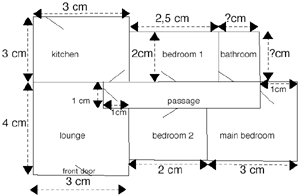SEARCH HOMEMath Central Quandaries & QueriesAbove is a plan of a house. The scale is 1 cm = 0,8 metres. 3.1 Describe briefly the rooms you come to as you go through the front door and down the passage. Say whether each room is on your left, right, ahead, etc 3.2 Find the actual dimensions of the bathroom (length and breadth) 3.3 Give the length of the passage in metres. 3.4 Give the area of a carpet needed to cover the passage 3.5 A child is in the lounge, at the door into the passage. He pushes a toy car, and it take 2 seconds to go from one end of the passage to the other. Calculate the speed at which the car travels, first in metres per second, then in kilometres per hour. Give the answer correct to 1 decimal place.Hi,

Here is the plan you sent.I can get you started by finding the actual dimensions of bedroom 1. This bedroom, on the plan, in 2 cm by 2,5 cm. The scale is 1 cm = 0,8 metres so 2 cm is 2 × 0,8 = 1,6 metres and 2,5 cm is 2,5 × 0,8 = 2,0 metres. Thus bedroom 1 is 1,6 metres by 2,0 metres.

PennyMath Central is supported by the University of Regina and The Pacific Institute for the Mathematical Sciences.